A pressure P - absolute temperature T diagram was obtained when a given mass of gas was heated. During the heating process from the state 1 to state 2 the volume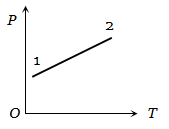1. Remained constant

2. Decreased

3. Increased

4. Changed erratically

High Yielding Test Series + Question Bank - NEET 2020

Difficulty Level:

A volume V and pressure P diagram was obtained from state 1 to state 2 when a given mass of a gas is subjected to temperature changes. During this process the gas is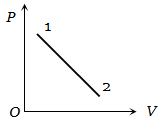1. Heated continuously

2. Cooled continuously

3. Heated in the beginning and cooled towards the end

4. Cooled in the beginning and heated towards the end

High Yielding Test Series + Question Bank - NEET 2020

Difficulty Level:

The figure shows the volume V versus temperature T graphs for a certain mass of a perfect gas at two constant pressures of ${\mathrm{P}}_{1}$ and ${\mathrm{P}}_{2}$ . What interference can you draw from the graphs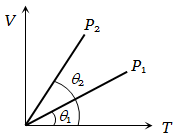1.

2.

3.  ${\mathrm{P}}_{1}={\mathrm{P}}_{2}$

4. No interference can be drawn due to insufficient information

High Yielding Test Series + Question Bank - NEET 2020

Difficulty Level:

The change in volume V with respect to an increase in pressure P has been shown in the figure for a non-ideal gas at four different temperatures  and ${\mathrm{T}}_{4}$ . The critical temperature of the gas is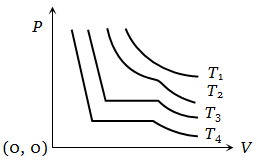1. ${\mathrm{T}}_{1}$

2. ${\mathrm{T}}_{2}$

3. ${\mathrm{T}}_{3}$

4. ${\mathrm{T}}_{4}$

High Yielding Test Series + Question Bank - NEET 2020

Difficulty Level:

In the adjoining figure, various isothermals are shown for a real gas. Then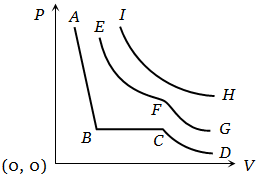1. EF represents liquification

2. CB represents liquification

3. HI represents the critical temperature

4. AB represents gas at a high temperature

High Yielding Test Series + Question Bank - NEET 2020

Difficulty Level:

From the following P-T graph what interference can be drawn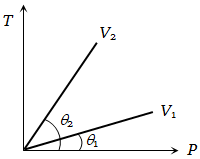1.

2.

3.

4. None of the above

High Yielding Test Series + Question Bank - NEET 2020

Difficulty Level:

PV versus T graph of equal masses of ${\mathrm{H}}_{2}$$\mathrm{He}$ and ${\mathrm{O}}_{2}$ is shown in fig. Choose the correct alternative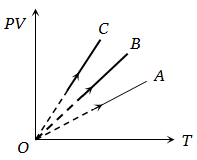1. C corresponds to ${\mathrm{H}}_{2}$, B to $\mathrm{He}$ and A to ${\mathrm{O}}_{2}$

2. A corresponds to $\mathrm{He}$, B to ${\mathrm{H}}_{2}$ and C to ${\mathrm{O}}_{2}$

3. A corresponds to $\mathrm{He}$, B to ${\mathrm{O}}_{2}$ and C to ${\mathrm{H}}_{2}$

4. A corresponds to ${\mathrm{O}}_{2}$, B to ${\mathrm{H}}_{2}$ and C to ${\mathrm{H}}_{2}$

High Yielding Test Series + Question Bank - NEET 2020

Difficulty Level:

Pressure versus temperature graph of an ideal gas at constant volume V of an ideal gas is shown by the straight line A. Now mass of the gas is doubled and the volume is halved, then the corresponding pressure versus temperature graph will be shown by the line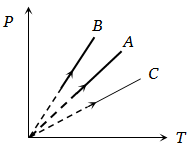1. A

2. B

3. C

4. None of these

High Yielding Test Series + Question Bank - NEET 2020

Difficulty Level:

Two different isotherms representing the relationship between pressure P and volume V at a given temperature of the same ideal gas are shown for masses ${\mathrm{m}}_{1}$ and ${\mathrm{m}}_{2}$ of the gas respectively in the figure given, then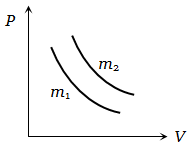1.

2.

3.

4. None of these

High Yielding Test Series + Question Bank - NEET 2020

Difficulty Level:

Two different masses m and 3m of an ideal gas are heated separately in a vessel of constant volume, the pressure P and absolute temperature T, graphs for these two cases are shown in the figure as A and B. The ratio of slopes of curves B to A is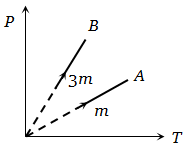1.  3 : 1

2.  1 : 3

3.  9 : 1

4.  1 : 9